+

# Area

Author: Sophia Tutorial
##### Description:

Calculate the area of a rectangle, square, and circle using formulas for area.

(more)

Sophia’s self-paced online courses are a great way to save time and money as you earn credits eligible for transfer to many different colleges and universities.*

No credit card required

37 Sophia partners guarantee credit transfer.

299 Institutions have accepted or given pre-approval for credit transfer.

* The American Council on Education's College Credit Recommendation Service (ACE Credit®) has evaluated and recommended college credit for 33 of Sophia’s online courses. Many different colleges and universities consider ACE CREDIT recommendations in determining the applicability to their course and degree programs.

Tutorial
what's covered
1. Introduction
2. Common Area Formulas
3. Calculate Area Given Side Lengths or Radii
4. Calculate Side Lengths or Radii Given Area

# 1. Introduction

When we try to determine how much space a is covered in a two-dimensional space, we need to use the concept of area. For example, when buying a house we oftentimes will consider the total living space in a house, which is considering how much floor space the house has.

Because area is represented in two-dimensions, we represent quantities of area as squared units. For example, we might say that a square living room has a total area of 625 square feet, which might mean that the length of the room is 25 feet and the width is 25 feet.

brainstorm
Can you think of another example where we might need to use area?

Suppose we wanted to paint the outer walls of a house, we may want to estimate the total area of the house’s exterior to get an estimate of the total amount of paint that we would need.

big idea
You will notice that when calculating area you will always be multiplying two distances with one another to determine the two dimensional space covered by an object.

# 2. Common Area Formulas

When calculating areas, there are a few common shapes that you will always come across. These shapes and their area formulas are listed below.

formula
Area of a Rectangle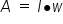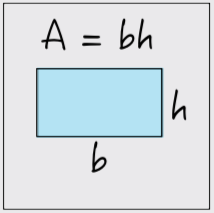Note that the area of a square is a special case of the area of a rectangle formula where the length and width are the same.

formula
Area of a Triangle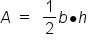Note that the height of a triangle is the distance of the line from one vertex (or corner) of the triangle to the opposite base, such that the line is perpendicular to the base.

formula
Area of a Circle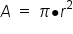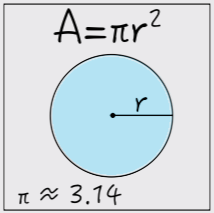Note that the radius of a circle is the distance from the center of a circle to the edge of the circle. Pi is a constant irrational number equal to 3.14159265….

Let’s look at how we can use these different area formulas to find some unknown quantity.

# 3. Calculate the Area Given Side Lengths or Radii

EXAMPLE

Suppose we are told that a rectangle has a length of 10 feet and a width of 12 feet. How would we find the rectangle’s area?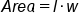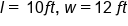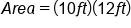Multiply length by width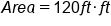Units of feet are also multiplied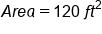Our Solution

Notice that when calculating area, we square the units of distance.

EXAMPLE

Suppose we are asked to find the area of a circle with a diameter of 9 inches. How would we make this calculation? As with the previous example, we start by writing down the appropriate area formula, and substitute in the quantities we know.

Here, we are given the diameter of the circle, but we need to know the radius to use the formula. The diameter of a circle is simply the distance of the line passing through the center of a circle and touching the circle’s edge. In other words the diameter, d, is twice as long as the radius, r, (d= 2r).

Since the diameter for this circle was 9 inches, the radius must be 4.5 inches.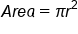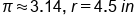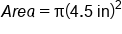Substitute 4.5 in for r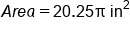Square the radius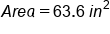Our Solution, rounded to the tenths place

# 4. Calculate Side Lengths or Radii Given Area

Sometimes we may be given the area of an object and need to back solve to find the measurements of a given part of an object. Let’s look at some examples.

EXAMPLE

If the area of a square is 400 square feet what is the length of the square?

When solving these types of problems, we follow the same process as we did before but then do some algebraic manipulation to solve the problem. Note that the length and width of a square is the same so we can refer to side length using a single variable, s.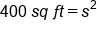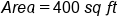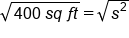Take the square root of both sides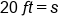Our Solution

EXAMPLE

If the area of a circle is 100pi ft^2, what is the radius of the circle?

Like in Example 3, we begin by substituting what we know into the appropriate formula. Notice that the area contains pi in it. This is often times the same when we represent area exactly; we leave the pi multiplied to the number.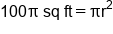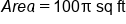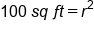Divide both sides by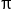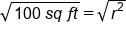Take the square root of both sides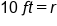Our Solution

summary
As an introduction to area, we learned that area of an object is the amount of space enclosed in a two dimensional shape. Area is measured in square units, such as centimeters squared or inches squared. Common area formulas include areas of rectangles, triangles, and circles. We can also use formulas to calculate the area when given side lengths or radii, as well as to calculate the side lengths or radii when given an area.

Terms to Know
pi

The ratio of a circle's circumference to its diameter; approximately 3.14.

Formulas to Know
Area of CircleArea of RectangleArea of TriangleRating